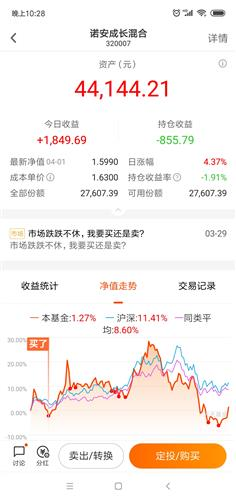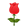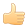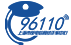• 最近访问：10+20+40+80+160+320+640+1280+2560+5120=10230（十次接刀机会，可接受10*5%=50%的回调）
100+200+300+400+500+600+700+800+900+1000+1100+1200+1300+1400=10500
（十四次接刀机会，可接受14*5%=70%的回调）
500*20=10000（二十次接刀机会，可接受20*5%=100%的回调）
200*50=10000（五十次接刀机会，可接受50*5%=250%的回调）